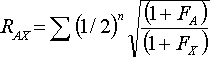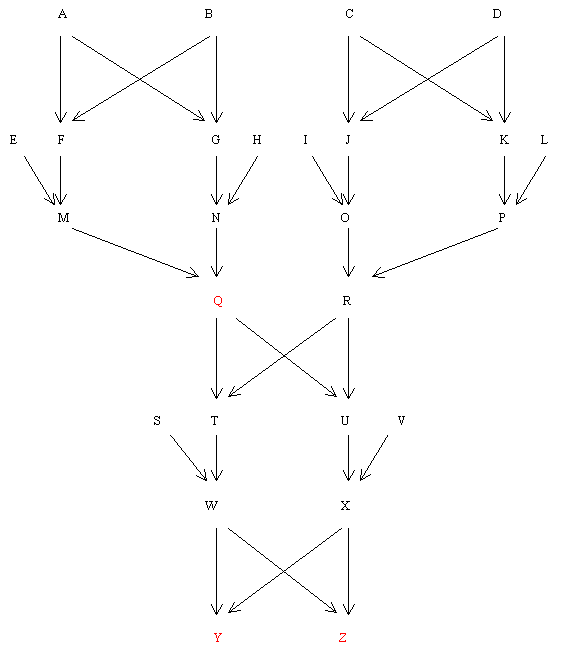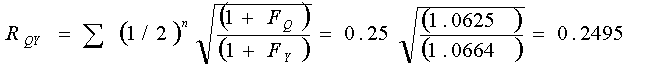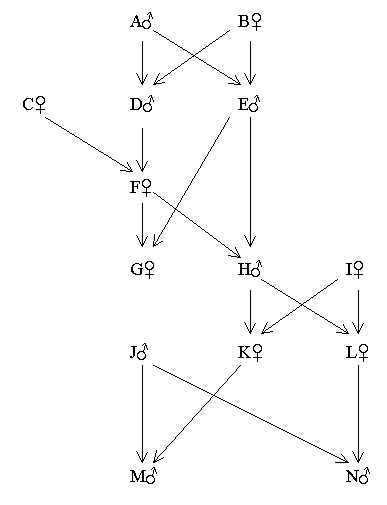## Appendix A - EXTENDED FORMULAE FOR THE coefficient of relationship

The Effects of Inbreeding on the Coefficient of Relationship

The main effect of inbreeding is to increase the coefficient of relationship (R), above its standard value, by creating double or multiple relationships. It does this in two ways:

·          By raising the number of connecting pathways between the relatives concerned; and

·          By increasing the number of common ancestors shared by collateral relatives.

Fortunately, the regular formula, R = Σ(1/2)n, allows for these increases.

Inbreeding also affects R in a more subtle way, which is not catered for by the above formula. These additional influences, which are described below, depend on whether the relationship is direct or collateral and also on which of the relatives are inbred.

Direct Relationship

Under normal circumstances the proportion of genes transmitted from ancestor to descendant [as estimated by Σ(1/2)n] and the proportion of genes they hold in common (the true coefficient of relationship) are the same. If there has been any inbreeding, however, there may be a discrepancy.

Situation A

If only the ancestor is inbred then Σ(1/2)n is an underestimate of the true coefficient of relationship (R) between ancestor and descendant. Σ(1/2)n, which can never exceed 50% unless the descendant is inbred, will be lower than the proportion of genes they hold in common at a particular locus. Because of previous inbreeding the ancestor may have two identical genes at that locus, whereas the descendant (not being inbred) is more likely to have only one, giving a total proportion of common inheritance at that locus of 75% (see here).

Situation B

Conversely, if only the descendant is inbred then Σ(1/2)n is an overestimate of R. This time, Σ(1/2)n for the relevant locus could be 100%, because both the descendant's genes are likely to have come from the same ancestor, but because the ancestor is not inbred, the proportion of genes they have in common is still only 75% (see here ).

To correct for the above errors the following extended formula should be used:

Equation 2Where FA and FX are the coefficients of inbreeding of the ancestor and the descendant respectively.

Collateral Relationship

In the case of collateral relatives, no genes are transmitted from one to the other, but are received from a common source. Thus, Σ(1/2)n measures the likely proportion of genes received from common ancestors which would normally be shared by the two collaterals. However, if there has been any inbreeding it may not be equal to R for the following reasons:

Situation C

If the common ancestor is inbred but neither of the collateral relatives, Σ(1/2)n will give an underestimate of common inheritance (R). Because the common ancestor has a higher degree of homozygosity than normal, he or she is more likely to transmit the same gene to both collaterals; thus raising their common inheritance above the standard value.

Situation D

In the opposite situation where both collaterals are inbred, but not the common ancestor, the likelihood that they will receive the same genes from that common ancestor is overestimated. Inbred collaterals diverge more quickly from each other than if they had not been inbred. This is because the fixation of genes (increase in homozygosity) is random with respect to each locus, so that inbred collaterals will tend to become homozygous for different alleles (i.e. AA or aa). This will reduce their coefficient of relationship below Σ(1/2)n.

In both cases the second extended formula restores R to its true value:

Equation 3Where FA is the inbreeding coefficient of the common ancestor, and FX and FY are the coefficients of the two collateral relatives.

 Examples

Number 1 is a fully worked example. Although numbers 2. 3 and 4 only give summarised results, they do indicate all the double and multiple relationships generated by inbreeding.

The pedigree shown below is an example of mild inbreeding. Because the inbreeding coefficients have to be calculated first, the whole process has to be divided into several stages. The first example shows how to calculate R for both direct and collateral relatives and is split into four stages.

Objective:- To find RQY and RYZ.

Q and Y have a direct relationship and Y and Z are collaterally related. It is, therefore, necessary to find FQ and FY before calculating RQY. The only inbred common ancestors of Y and Z are Q and R, but since FQ = FR and FY = FZ (identical pedigrees), RYZ can also be calculated from the same data.

Figure 76 Pedigree Involving Three First Cousin MarriagesStage 1 Find FQ

 Common ancestorsof parents M and N Paths (1/2)n+1 (1 + FA) A M_1_F_2_A_3_G_4_N 1/32 x 1.0 = 0.03125 B M_1_F_2_B_3_G_4_N 1/32 x 1.0 = 0.03125 Σ[(1/2)n+1 x (1 + FA)] = 0.06250

Therefore FQ ( = FR) = 0.0625

Stage 2 Find FY

 Common ancestorsof Parents W and X Paths (1/2)n+1 x (1 + FA) Q W_1_T_2_Q_3_U_4_X 1/32 x 1.0625 = 0.033203 R W_1_T_2_R_3_U_4_X 1/32 x 1.0625 = 0.033203 Σ[(1/2)n+1 (1 + FA)] = 0.066406

Therefore FY ( = FZ) = 0.0664

Stage 3 Find RQY (Direct relationship)

 Paths (1/2)n Q_1_T_2_W_3_Y 1/8 = 0.125 Q_1_U_2_X_3_Y 1/8 = 0.125 Σ(1/2)n = 0.250

Equation 4Uncorrected Σ(1/2)n = 0.25

Stage 4 Find RYZ (Collateral relationship)

 Common ancestors Paths (1/2)n x (1 + FA) W Y_1_W_2_Z 1/4 x 1.0 = 0.25 X Y_1_X_2_Z 1/4 x 1.0 = 0.25 Q Y_1_W_2_T_3_Q_4_U_5_X_6_Z 1/16 x 1.0625 = 0.066406 Y_1_X_2_U_3_Q_4_T_5_W_6_Z R Y_1_W_2_T_3_R_4_U_5_X_6_Z Y_1_X_2_U_3_R_4_T_5_W_6_Z Σ[(1/2)n (1 + FA)] = 0.566406

Equation 5Uncorrected Σ(1/2)n = 0.5625

With mild inbreeding it can be seen that the errors are not great when using [R = Σ(1/2)n], and are probably less than the sampling errors which occur naturally.

#### Example 2 - Forbidden relationships (uncle - niece)

Children from forbidden marriage relationships, with higher coefficients of inbreeding, will lead to larger errors. Although an uncle-niece marriage is forbidden, any children produced would not be regarded as incestuous (see here).

Figure 77 Pedigree Involving a Forbidden Uncle-niece RelationshipThe only inbred persons in the above pedigree are G and H. (FG = FH = 12.5%)

Corrections for Inbreeding (Example 2 continued)

 Increased Relationships (Ancestors inbred) UncorrectedΣ(1/2)n (%) R (Corrected for inbreeding) (%) Direct RHM (Grandfather-grandson) 25.0 26.5 RHL (Father-daughter) 50.0 53.0 Collateral RKL (Full sibs) 50.0 53.1 RMN Half sibs (25.0%) 37.5 38.3 Single first cousins (12.5%) Reduced Relationships (Descendants inbred) Direct RCH (Grandmother-grandson) 25.0 23.6 RAH Grandfather-grandson (25.0%) 37.5 35.4 Great-grandfather great-grandson (12.5%) Collateral RGH Full sibs (50.0%) 62.5 55.6 Double (first cousins once removed) (12.5%) Combined RDH Grandfather-grandson (25.0%) 50.0 47.1 Uncle-nephew (25.0%) RFH Mother-son (50.0%) 62.5 58.9 Single first cousins (12.5%) REH Father-son (50.0%) 62.5 58.9 Great uncle-great nephew (12.5%)

Conclusions

The errors are now starting to increase. Although still not particularly high it would be prudent in this instance to use the extended formulae.

Example 3 - Incest (father - daughter, brother - sister)

Figure 78 Father-daughter and Brother-sisterFather-Daughter Uncorrected Σ(1/2)n (%) R (Corrected for inbreeding) (%) RAD (Grandmother-grandson) 25.0 22.4 RBD Father-son (50%) 75.0 67.1 Grandfather-grandson (25.0%) RCD Mother-son (50.0%) 75.0 67.1 Half sister-half brother (25%) Brother-Sister RAE (Double grandfather-grandson relationship) 50.0 44.7 RBE (Double grandmother-grandson relationship) 50.0 44.7 RCE Father-son (50.0%) 75.0 67.1 Uncle-nephew (25.0%) RDE Mother-son (50.0%) 75.0 67.1 Aunt-nephew (25.0%)

Conclusion

In this case the basic formula leads to significant errors and, therefore, the extended formulae should be used.

Figure 79 Sib Relationship Following Three Generations of Full Sib MatingFind RIJ

 Uncorrected Σ(1/2)n (%) R (Corrected for inbreeding) (%) RIJ Brother-sister (50%) 93.75 79.17 Double first cousins (25%) Quadruple second cousins (12.5%) Octuple third cousins (6.25%

Conclusion

I and J have a complicated, mixed multiple relationship. The large error of 14.6% shows that it is essential to use the extended formula for such cases.